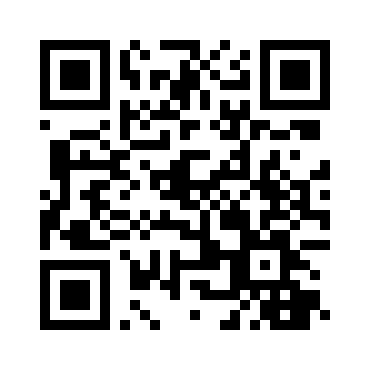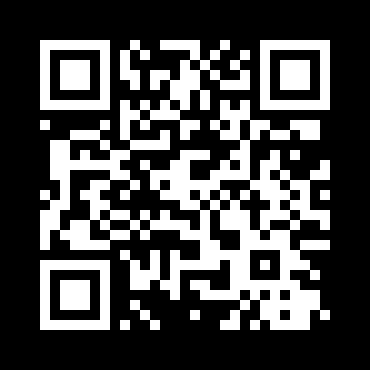# How to Generate and Read QR Code in Python

Learning how you can generate and read QR Code in Python using qrcode and OpenCV libraries.
6 min read · Updated may 2022 · Computer Vision · Python for Multimedia

Kickstart your coding journey with our Python Code Assistant. An AI-powered assistant that's always ready to help. Don't miss out!

Disclosure: This post may contain affiliate links, meaning when you click the links and make a purchase, we receive a commission.

QR code is a type of matrix barcode that is a machine-readable optical label that contains information about the item to which it is attached. In practice, QR codes often contain data for a locator, identifier, or tracker that points to a website or application, etc.

In this tutorial, you will learn how to generate and read QR codes in Python using qrcode and OpenCV libraries.

Installing required dependencies:

``pip3 install opencv-python qrcode numpy``

## Generate QR Code

First, let's start by generating QR codes, it is basically straightforward using qrcode library:

``````import qrcode
# example data
data = "https://www.thepythoncode.com"
# output file name
filename = "site.png"
# generate qr code
img = qrcode.make(data)
# save img to a file
img.save(filename)``````

This will generate a new image file in the current directory with the name of "site.png", which contains a QR code image of the data specified (in this case, this website URL), will look something like this:You can also use this library to have full control with QR code generation using the `qrcode.QRCode()` class, in which you can instantiate and specify the size, fill color, back color, and error correction, like so:

``````import qrcode
import numpy as np
# data to encode
data = "https://www.thepythoncode.com"
# instantiate QRCode object
qr = qrcode.QRCode(version=1, box_size=10, border=4)
# add data to the QR code
# compile the data into a QR code array
qr.make()
# print the image shape
print("The shape of the QR image:", np.array(qr.get_matrix()).shape)
# transfer the array into an actual image
img = qr.make_image(fill_color="white", back_color="black")
# save it to a file
img.save("site_inversed.png")``````

So in the creation of `QRCode` class, we specify the `version` parameter, which is an integer from 1 to 40 that controls the size of the QR code image (1 is small, 21x21 matrix, 40 is 185x185 matrix), but this will be overwritten when the data doesn't fit the size you specify. In our case, it will scale up to version 3 automatically.

`box_size` parameter controls how many pixels each box of the QR code is, whereas the `border` controls how many boxes thick the border should be.

We then add the data using the `qr.add_data()` method, compiles it to an array using `qr.make()` method, and then make the actual image using `qr.make_image()` method. We specified white as the `fill_color` and black as the `back_color`, which is the exact opposite of the default QR code, check it out:And the shape of the image was indeed scaled up and wasn't 21x21:

``The shape of the QR image: (37, 37)``

There are many tools that read QR codes. However, we will be using OpenCV for that, as it is popular and easy to integrate with the webcam or any video.

Alright, open up a new Python file and follow along with me, let's read the image that we just generated:

``````import cv2

Luckily for us, OpenCV already got QR code detector built-in:

``````# initialize the cv2 QRCode detector
detector = cv2.QRCodeDetector()``````

We have the image and the detector, let's detect and decode that data:

``````# detect and decode
data, bbox, straight_qrcode = detector.detectAndDecode(img)``````

The detectAndDecode() function takes an image as an input and decodes it to return a tuple of 3 values: the data decoded from the QR code, the output array of vertices of the found QR code quadrangle, and the output image containing rectified and binarized QR code.

We just need data and bbox here, bbox will help us draw the quadrangle in the image and data will be printed to the console!

Let's do it:

``````# if there is a QR code
if bbox is not None:
print(f"QRCode data:\n{data}")
# display the image with lines
# length of bounding box
n_lines = len(bbox)
for i in range(n_lines):
# draw all lines
point1 = tuple(bbox[i])
point2 = tuple(bbox[(i+1) % n_lines])
cv2.line(img, point1, point2, color=(255, 0, 0), thickness=2)``````

cv2.line() function draws a line segment connecting two points, we retrieve these points from bbox array that was decoded by detectAndDecode() previously. we specified a blue color ( (255, 0, 0) is blue as OpenCV uses BGR colors ) and thickness of 2.

Finally, let's show the image and quit when a key is pressed:

``````# display the result
cv2.imshow("img", img)
cv2.waitKey(0)
cv2.destroyAllWindows()``````

Once you run this, the decoded data is printed:

``````QRCode data:
https://www.thepythoncode.com``````

And the following image is shown:

``

As you can see, the blue lines are drawn in the exact QR code borders. Awesome, we are done with this script, try to run it with different data and see your own results!

Note that this is ideal for QR codes and not for barcodes, If you want to read barcodes, check this tutorial that is dedicated to that!

If you want to detect and decode QR codes live using your webcam (and I'm sure you do), here is a code for that:

``````import cv2
# initalize the cam
cap = cv2.VideoCapture(0)
# initialize the cv2 QRCode detector
detector = cv2.QRCodeDetector()
while True:
# detect and decode
data, bbox, _ = detector.detectAndDecode(img)
# check if there is a QRCode in the image
if bbox is not None:
# display the image with lines
for i in range(len(bbox)):
# draw all lines
cv2.line(img, tuple(bbox[i]), tuple(bbox[(i+1) % len(bbox)]), color=(255, 0, 0), thickness=2)
if data:
print("[+] QR Code detected, data:", data)
# display the result
cv2.imshow("img", img)
if cv2.waitKey(1) == ord("q"):
break
cap.release()
cv2.destroyAllWindows()``````

Awesome, we are done with this tutorial, you can now integrate this into your own applications!

Finally, if you're a beginner and want to learn Python, I suggest you take the Python For Everybody Coursera course, in which you'll learn a lot about Python, good luck!

Learn also:

Happy Coding ♥

Found the article interesting? You'll love our Python Code Generator! Give AI a chance to do the heavy lifting for you. Check it out!

Sharing is caring!

### Comment panel

Got a coding query or need some guidance before you comment? Check out our Python Code Assistant for expert advice and handy tips. It's like having a coding tutor right in your fingertips!